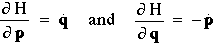# Classical Mechanics

This is the conventional, "intuitive" mechanics. There are three main type of mechanics, which differ in how they write down the characteristic equations of motion.

### Newtonian mechanics

This the most common and well-known class of mechanics, based on Newton's Equations of Motion: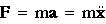Notice that this is a second order differential equation. Given two initial conditions, such as particle position and particle velocity at time zero, all subsequent states of the system, i.e. positions and velocities and all quantities which are functions of these, may be solved for deterministically. For a system of N particles, this corresponds to 3N 2nd order ODE's, and requires 6N initial conditions for its solution (in 3 dimensions).

The total energy of a system of particles depends upon the positions and velocities of those particles and may be broken down into a kinetic energy, which depends only on the particle velocities, and a potential energy, which depends on particle positions.We will only be concerned with Conservative Systems, in which we can express the forces on the particles in terms of the gradient of potential energy: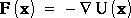or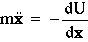In Newtonian mechanics, a change in kinetic energy K is directly related to a change in the potential energy U, and the total energy E is conserved.

### Lagrangian Mechanics

Newtonian mechanics, while simple in Cartesian coordinates, is not invariant to transformation to other coordinate systems (e.g. cylindrical, polar). Lagrange's equations of motion are obtained by differentiation of the so-called Lagrangian L, defined as:

L = K - U

A big advantage of Lagrange's equations of motion is that the form is invariant to transformation from one coordinate system to another. We can introduce a set of generalized coordinates q and the corresponding generalized velocities q. The we can write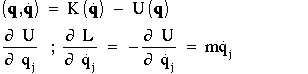The equation F=ma now becomes: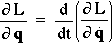which is the Lagrangian equation of motion. This too is a 2nd order ODE. For a system of N particles we have 3N equations and 6N initial conditions (in 3 dimensions).

### Hamiltonian Mechanics

Whereas Newtonian and Lagrangian mechanics are formulated in terms of particle positions and velocities, Hamiltonian mechanics are formulated in terms of particle positions and momenta. Hamiltonian mechanics retains invariance to coordinate transformation, hence these are generalized positions (q) and momenta (p).

Generalized momenta are defined as those variables conjugate to the generalized coordinates (positions) in Lagrangian mechanics.

definition: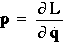For example, in Cartesian coordinates (x,y) the generalized momenta are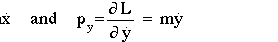while in polar coordinates (r, theta) the generalized momenta areThe Hamiltonian H is defined asTo get the Hamiltonian equations of motion, we want to differentiate H(p,q) with respect to p and q. This leads to 6N 1st order ODE's for a system of N particles in 3 dimensions. 6N initial conditions are still required.

If we have potential energy U=U(q), a function of position only, and kinetic energy K=K(q), a function of velocities only, and furthermore K is quadratic in q, such as K(q)=aq2, then the momentum p is simply: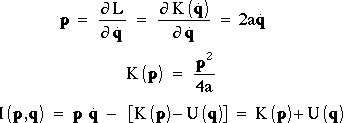Differentiating H(p,q) with respect to p and q gives the following Hamiltonian Equations of Motion: